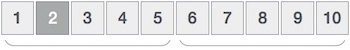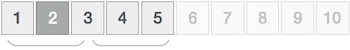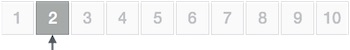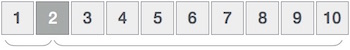# Data Structure - Interpolation Search

Interpolation search is an improved variant of binary search. This search algorithm works on the probing position of the required value. For this algorithm to work properly, the data collection should be in a sorted form and equally distributed.

Binary search has a huge advantage of time complexity over linear search. Linear search has worst-case complexity of Ο(n) whereas binary search has Ο(log n).

There are cases where the location of target data may be known in advance. For example, in case of a telephone directory, if we want to search the telephone number of Morphius. Here, linear search and even binary search will seem slow as we can directly jump to memory space where the names start from 'M' are stored.

## Positioning in Binary Search

In binary search, if the desired data is not found then the rest of the list is divided in two parts, lower and higher. The search is carried out in either of them.Even when the data is sorted, binary search does not take advantage to probe the position of the desired data.

## Position Probing in Interpolation Search

Interpolation search finds a particular item by computing the probe position. Initially, the probe position is the position of the middle most item of the collection.If a match occurs, then the index of the item is returned. To split the list into two parts, we use the following method −

```mid = Lo + ((Hi - Lo) / (A[Hi] - A[Lo])) * (X - A[Lo])

where −
A    = list
Lo   = Lowest index of the list
Hi   = Highest index of the list
A[n] = Value stored at index n in the list
```

If the middle item is greater than the item, then the probe position is again calculated in the sub-array to the right of the middle item. Otherwise, the item is searched in the subarray to the left of the middle item. This process continues on the sub-array as well until the size of subarray reduces to zero.

Runtime complexity of interpolation search algorithm is Ο(log (log n)) as compared to Ο(log n) of BST in favorable situations.

### Algorithm

As it is an improvisation of the existing BST algorithm, we are mentioning the steps to search the 'target' data value index, using position probing −

```Step 1 − Start searching data from middle of the list.
Step 2 − If it is a match, return the index of the item, and exit.
Step 3 − If it is not a match, probe position.
Step 4 − Divide the list using probing formula and find the new midle.
Step 5 − If data is greater than middle, search in higher sub-list.
Step 6 − If data is smaller than middle, search in lower sub-list.
Step 7 − Repeat until match.
```

## Pseudocode

```A → Array list
N → Size of A
X → Target Value

Procedure Interpolation_Search()

Set Lo  →  0
Set Mid → -1
Set Hi  →  N-1

While X does not match

if Lo equals to Hi OR A[Lo] equals to A[Hi]
end if

Set Mid = Lo + ((Hi - Lo) / (A[Hi] - A[Lo])) * (X - A[Lo])

if A[Mid] = X
EXIT: Success, Target found at Mid
else
if A[Mid] < X
Set Lo to Mid+1
else if A[Mid] > X
Set Hi to Mid-1
end if
end if
End While

End Procedure
```